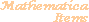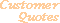Home Older Mathematica Items

Scientific Arts
` `

# Eye Diagram for Digital Communications

Page 1 | Page 2

## Introduction

In digital communications the "eye diagram" is used to visualize how the waveforms used to send multiple bits of data can potentially lead to errors in the interpretation of those bits. This is the so-called problem of intersymbol interference. Certain pulse shapes are used specifically to alleviate this. One simple example of this is the "raised cosine" pulse shape. Here we give an example of an implementation of the eye diagram for the raised cosine pulse shape for Pulse Amplitude Modulation (PAM) waveforms.

## Binary Pulse Amplitude Modulation

Define the raised cosine pulse shape.

``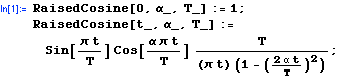``

Here is a plot of the raised cosine pulse for several values of the parameter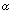.

``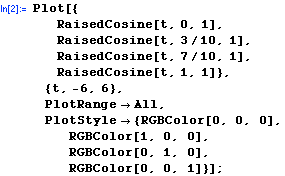``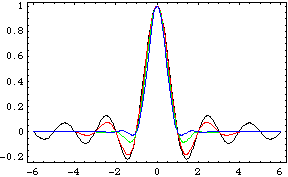Now define a function that gives a random binary datum.

````

A PAM (Pulse Amplitude Modulated) waveform can be built by combining the pulse shaping factor (in our case the raised cosine pulse) with a coefficient that depends on the bits being transmitted.

For a baseband BPAM (Binary Pulse Amplitude Modulated) waveform the coefficients are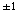depending on whether a bit is 1 or 0. Here is a function that creates the BPAM waveform corresponding to a user supplied bit sequence.

``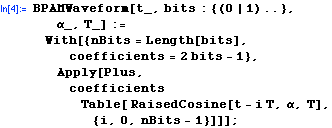``

Here is a version where a random bit sequence is generated that is nBits long.

``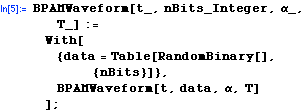` `
 This could also have been written as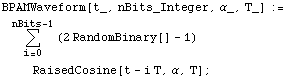Here is the waveform for a random sequence of 20 bits (which we call dataTest) as a function of time.

``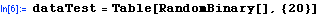``
`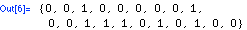`
````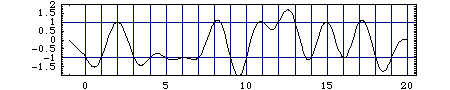The eye diagram is constructed by overlaying plots of the waveform from successive unit time intervals. This is a function that makes an eye diagram from a random bit sequence that is nBits long.

``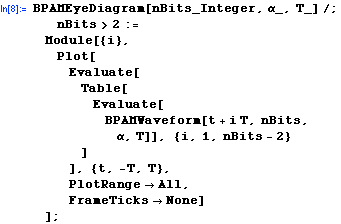``

And here is the version that takes a user supplied bit sequence.

``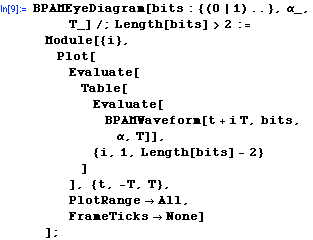``

Here is an example of the eye diagram.

````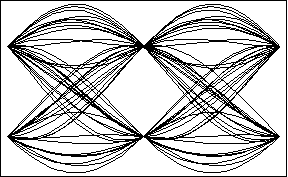Let's look at the eye diagram for different values of theparameter in the raised cosine pulse. From the frequency domain representation of the raised cosine pulse it is known that asvaries from 0 to 1 the excess bandwidth required varies fromto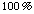.  First generate a random bit sequence.

````
`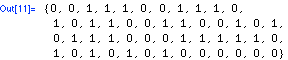`

Now show the eye diagrams for this particular bit sequence for.

``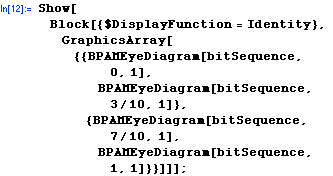``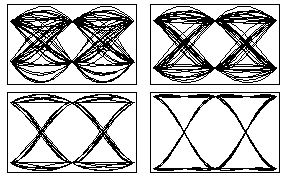The eye diagram becomes much more "open" (and hence the transmission of the data less prone to errors due to noise, timing drift, etc...) asgets closer to 1.  Of course the penalty that one has to pay for this is the increase in the needed bandwidth.

## Generalization to Higher Order Pulse Amplitude Modulation

 For further information on our services send email to info@scientificarts.com . Contents of this web site Copyright © 1999-2011, Scientific Arts, LLC.
 s